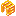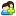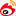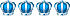【高优指导】2017版高考数学一轮复习 第十二章 推理与证明、算法初步与复数 12.2 综合法、分析法、反证法课件 文 北师大版 版权投诉1 该PPT不包含附件（如视频、讲稿)，本站只保证下载后内容跟在线阅读一样，不确保内容完整性，请务必认真阅读

2 有的文档阅读时显示本站（www.woc88.com）水印的，下载后是没有本站水印的（仅在线阅读显示），请放心下载

3 除PDF格式下载后需转换成word才能编辑，其他下载后均可以随意编辑修改

4 有的标题标有”最新”、多篇，实质内容并不相符，下载内容以在线阅读为准，请认真阅读全文再下载

5 该文档为会员上传，下载所得收益全部归上传者所有，若您对文档版权有异议，可联系客服认领，既往收入全部归您

1 点击【下载】按钮，注册或登陆账号 2 登陆后再点【下载】按钮，在弹出的窗口选择下载方式，扫码付款
3 付款后关掉下载提示，重新点【下载】按钮 4 在弹出的窗口，点【下载文档到电脑】，便可以在电脑打开编辑了文档类型：PPT 文档上传时间：2020-04-20 15:47:19
0精品推荐《【高优指导】2017版高考数学一轮复习 第十二章 推理与证明、算法初步与复数 12.2 综合法、分析法、反证法课件 文 北师大版.ppt》由会员分享，可在线阅读全文，更多相关《【高优指导】2017版高考数学一轮复习 第十二章 推理与证明、算法初步与复数 12.2 综合法、分析法、反证法课件 文 北师大版》请在www.woc88.com上搜索。

1、,往往采用分析法,特别是含有根号、绝对值的等式或不等式,从正面不易推导时,常考虑用分析法考点考点考点知识方法易错易混对点训练已知a≥bgt,求证:ab≥abab答案答案关闭证明:要证明ab≥abab成立,只需证:abab+ab≥,即a(ab)+b(ab)≥,即(a+b)(ab)(a+b)≥∵a≥bgt,∴ab≥,a+bgt,a+bgt,从而(a+b)(ab)(a+b)≥成立,∴ab≥abab考点考点考点知识方法易错易混考点反证法的应用例设数列{an}是公比为q的等比数列,Sn是它的前n项和()求证:数列{Sn}不是等比数列()数列{Sn}是等差数列吗?为什么?答案答案关闭()证明:假设数列{Sn}是等比数列,则𝑆=SS,即𝑎。

2、,且推导出的矛盾必须是明显的考点考点考点知识方法易错易混应用分析法要书写规范,常用“要证”“只需证”等分析到一个明显成立的结论应用反证法要将假设作为条件进行推理,不使用假设而推出矛盾的,其推理过程是错误的注意推理的严谨性,在证明过程中每一步推理都要有充分的依据,这些依据就是命题的已知条件和已经掌握了的数学结论,不可盲目使用正确性未知的自造结论综合法、分析法、反证法考纲要求:了解直接证明的两种基本方法:分析法和综合法；了解分析法和综合法的思考过程和特点了解反证法的思考过程和特点综合法与分析法内容综合法分析法定义从命题的条件出发,利用定义、公理、定理及运算法则,通过演绎推理,一步一步地接近要证明的结论,直到完成命题的证明我们把这样的。分条件；综合法是由因导果,就是寻找已知的必要条件综合法和分析法都是直接证明的方法,反证法是间接证明的方法用反证法证题时,首先否定结论,否定结论就是找出结论的反面的情况然后推出矛盾,矛盾可以与已知、公理、定理、事实或者假设等相矛盾考点考点考点知识方法易错易混考点综合法的应用例设数列{an}的前n项和为Sn,且(m)Sn+man=m+(n∈N+)其中m为常数,且m≠()求证:{an}是等比数列；()若数列{an}的公比为q=f(m),数列{bn}满足b=a,bn=f(bn)(n∈N+,n≥),求证:𝑏𝑛为等差数列答案答案关闭证明:()由(m)Sn+man=m+,得(m)𝑆𝑛++m𝑎𝑛+=m+,两式相减,得(+m)𝑎𝑛+=man,m≠,∴𝑎𝑛+𝑎𝑛=𝑚𝑚+又m为常数,且m≠,∴{an}是等比数列()∵b=a=,q=f(m)=𝑚𝑚+,∴当n∈N+,且n≥时,bn=f(𝑏𝑛)=𝑏𝑛𝑏𝑛+⇒bnbn+bn=bn⇒𝑏𝑛−𝑏𝑛=∴𝑏𝑛是首项为,公差为的等差数列考点考点考点知识方法易知识不太明确、具体时。

3、ac)(ab)gt故求证𝑏𝑎𝑐答案解析关闭C(安徽亳州模拟)实数a,b,c满足a+b+c=,abcgt,则的值()A一定是正数B一定是负数C可能是D正、负不确定𝑎+𝑏+𝑐答案解析解析关闭由a+b+c=,abcgt得a,b,c中必有两负一正,不妨设a,且|a||𝑐|,从而𝑎gt𝑐而𝑏lt,所以𝑎+𝑏+𝑐lt答案解析关闭B在△ABC中,∠BAC,∠ABC,∠ACB的对边分别为a,b,c,若a,b,c三边的倒数成等差数列,则∠ABC(填“gt”“a,bgtc取倒数,得𝑎gt𝑏,𝑐gt𝑏,相加得𝑎+𝑐gt𝑏+𝑏=𝑏,这与𝑎+𝑐=𝑏矛盾故∠ABC≥不成立,即∠ABClt答案解析关闭lt自测。

4、错误的打“”()综合法的思维过程是由因导果,逐步寻找已知的必要条件()()分析法是从要证明的结论出发,逐步寻找使结论成立的充要条件()()用反证法证明时,推出的矛盾不能与假设矛盾()()常常用分析法寻找解题的思路与方法,用综合法展现解决问题的过程()()证明不等式最合适的方法是分析法()√+√lt√+√√√√命题“对于任意角θ,cosθsinθ=cosθ”的证明:“cosθsinθ=(cosθsinθ)(cosθ+sinθ)=cosθsinθ=cosθ”过程应用了()A分析法B综合法C综合法、分析法综合使用D间接证明法答案解析解析关闭因为证明过程是“从左往右”,即由条件⇒结论故选B答案解析关闭B用反证法证明命题“设a,b为实数,。n}是等差数列；当q≠时,{Sn}不是等差数列考点考点考点知识方法易错易混思考:反证法的适用范围及证题的关键是什么?解题心得:反证法的适用范围及证题的关键()适用范围:当一个命题的结论是以“至多”“至少”“唯一”或以否定形式出现时,宜用反证法来证()关键:在正确的推理下得出矛盾,矛盾可以是与已知条件矛盾,与假设矛盾,与定义、公理、定理矛盾,与事实矛盾等推导出的矛盾必须是明显的考点考点考点知识方法易错易混对点训练已知f(x)=ax+bx+c,若a+c=,f(x)在[,]上的最大值为,最小值为求证:a≠,且𝑏𝑎lt证明:假设a=,或𝑏𝑎≥()当a=时,由a+c=,得f(x)=bx,显然b≠由题意得f(x)=bx在[,]上是单调函数,所以f(x)的最大值为|b|,最小值为|b|由已知条件,得|b|+(|b|)==,这与|b|+(|b|)=相矛盾,所以a≠考点考点考点知识方法易错易混()当𝑏𝑎≥时,由二次函数的对称轴为x=𝑏𝑎,知f(x)在[,]上是单调函数,故其最值在区间的端点处取得所以𝑓()=𝑎+𝑏+𝑐=,𝑓()=𝑎。

5、C,∠ABC,∠ACB的对边分别为a,b,c,若a,b,c三边的倒数成等差数列,则∠ABC(填“gt”“a,bgtc取倒数,得𝑎gt𝑏,𝑐gt𝑏,相加得𝑎+𝑐gt𝑏+𝑏=𝑏,这与𝑎+𝑐=𝑏矛盾故∠ABC≥不成立,即∠ABClt答案解析关闭lt自测点评分析法是执果索因,实际上是寻找使结论成立的充分条件；综合法是由因导果,就是寻找已知的必要条件综合法和分析法都是直接证明的方法,反证法是间接证明的方法用反证法证题时,首先否定结论,否定结论就是找出结论的反面的情况然后推出矛盾,矛盾可以与已知、公理、定理、事实或者假设等相矛盾考点考点考点知识方法易错易混考点综合法的应用例设数列{an}的前n项和为Sn,且(m)Sn。

6、思维方法称为综合法从求证的结论出发,一步一步地探索保证前一个结论成立的充分条件,直到归结为这个命题的条件,或者归结为定义、公理、定理等我们把这样的思维方法称为分析法实质由因导果执果索因框图表示P⇒Q→Q⇒Q→→Qn⇒QQ⇐P→P⇐P→→得到一个明显成立的条件文字语言因为所以或由得要证只需证即证反证法()反证法的定义:在假定命题结论反面成立的前提下,经过推理,若推出的结果与定义、公理、定理矛盾,或与命题中的已知条件相矛盾,或与假定相矛盾,从而说明命题结论的反面不可能成立,由此断定命题结论成立的证明方法叫反证法()用反证法证题的步骤:①反设作出否定结论的假设；②归谬进行推理,导出矛盾；③结论否定假设,肯定结论下列结论正确的打“√”,。

7、(+q)=aa(+q+q),因为a≠,所以(+q)=+q+q,即q=,这与公比q≠矛盾,所以数列{Sn}不是等比数列()解:当q=时,Sn=na,故{Sn}是等差数列；当q≠时,{Sn}不是等差数列假设{Sn}是等差数列,则S=S+S,即a(+q)=a+a(+q+q),得q=,这与公比q≠矛盾综上,当q=时,数列{Sn}是等差数列；当q≠时,{Sn}不是等差数列考点考点考点知识方法易错易混思考:反证法的适用范围及证题的关键是什么?解题心得:反证法的适用范围及证题的关键()适用范围:当一个命题的结论是以“至多”“至少”“唯一”或以否定形式出现时,宜用反证法来证()关键:在正确的推理下得出矛盾,矛盾可以是与已知条件矛盾,与假设矛盾,。,往往采用分析法,特别是含有根号、绝对值的等式或不等式,从正面不易推导时,常考虑用分析法考点考点考点知识方法易错易混对点训练已知a≥bgt,求证:ab≥abab答案答案关闭证明:要证明ab≥abab成立,只需证:abab+ab≥,即a(ab)+b(ab)≥,即(a+b)(ab)(a+b)≥∵a≥bgt,∴ab≥,a+bgt,a+bgt,从而(a+b)(ab)(a+b)≥成立,∴ab≥abab考点考点考点知识方法易错易混考点反证法的应用例设数列{an}是公比为q的等比数列,Sn是它的前n项和()求证:数列{Sn}不是等比数列()数列{Sn}是等差数列吗?为什么?答案答案关闭()证明:假设数列{Sn}是等比数列,则𝑆=SS,即𝑎(+q)=aa(+q+q),因为a≠,所以(+q)=+q+q,即q=,这与公比q≠矛盾,所以数列{Sn}不是等比数列()解:当q=时,Sn=na,故{Sn}是等差数列；当q≠时,{Sn}不是等差数列假设{Sn}是等差数列,则S=S+S,即a(+q)=a+a(+q+q),得q=,这与公比q≠矛盾综上,当q=时,数列{S。

8、与定义、公理、定理矛盾,与事实矛盾等推导出的矛盾必须是明显的考点考点考点知识方法易错易混对点训练已知f(x)=ax+bx+c,若a+c=,f(x)在[,]上的最大值为,最小值为求证:a≠,且𝑏𝑎lt证明:假设a=,或𝑏𝑎≥()当a=时,由a+c=,得f(x)=bx,显然b≠由题意得f(x)=bx在[,]上是单调函数,所以f(x)的最大值为|b|,最小值为|b|由已知条件,得|b|+(|b|)==,这与|b|+(|b|)=相矛盾,所以a≠考点考点考点知识方法易错易混()当𝑏𝑎≥时,由二次函数的对称轴为x=𝑏𝑎,知f(x)在[,]上是单调函数,故其最值在区间的端点处取得所以𝑓()=𝑎+𝑏+𝑐=,𝑓()=𝑎。

9、果索因法,若用分析法证明:“已知agtbgtc,且a+b+c=,求证”索的因应是()AabgtBacgtC(ab)(ac)gtD(ab)(ac)bgtc,且a+b+c=可得b=ac,agt,c,即证a(ac)+(a+c)(ac)gt,即证a(ac)b(ac)gt,即证(ac)(ab)gt故求证𝑏𝑎𝑐答案解析关闭C(安徽亳州模拟)实数a,b,c满足a+b+c=,abcgt,则的值()A一定是正数B一定是负数C可能是D正、负不确定𝑎+𝑏+𝑐答案解析解析关闭由a+b+c=,abcgt得a,b,c中必有两负一正,不妨设a,且|a||𝑐|,从而𝑎gt𝑐而𝑏lt,所以𝑎+𝑏+𝑐lt答案解析关闭B在△ABC中,∠BA。𝑏+𝑐=,或𝑓()=𝑎+𝑏+𝑐=,𝑓()=𝑎𝑏+𝑐=又a+c=,则此时b无解,所以𝑏𝑎lt由()(),得a≠,且𝑏𝑎lt考点考点考点知识方法易错易混分析法是从结论出发,逆向思维,寻找使结论成立的充分条件应用分析法要严格按分析法的语言表达,下一步是上一步的充分条件证明问题的常用思路:在解题时,常常把分析法和综合法结合起来运用,先以分析法寻求解题思路,再用综合法表述解答或证明过程用反证法证明问题要把握三点:()必须先否定结论,即肯定结论的反面；()必须从否定结论进行推理,即应把结论的反面作为条件,且必须依据这一条件进行推证；()推导出的矛盾可能多种多样,有的与已知矛盾,有的与假设矛盾,有的与已知事实矛盾等,且推导出的矛盾必须是明显的考点考点考点知识方法易错易混应用分析法要书写规范,常用“要证”“只需证”等分析到一个明显成立的结论应用反证法要将假设作为条件进行推理,不使用假设而推出矛盾的,其推理过程是错误的注意推理的严谨性,在证明过程中每一步推理都要有充分的依据,这些依据就是命题的已知条件和已经掌握了的数学结论,不可盲。

10、+man=m+(n∈N+)其中m为常数,且m≠()求证:{an}是等比数列；()若数列{an}的公比为q=f(m),数列{bn}满足b=a,bn=f(bn)(n∈N+,n≥),求证:𝑏𝑛为等差数列答案答案关闭证明:()由(m)Sn+man=m+,得(m)𝑆𝑛++m𝑎𝑛+=m+,两式相减,得(+m)𝑎𝑛+=man,m≠,∴𝑎𝑛+𝑎𝑛=𝑚𝑚+又m为常数,且m≠,∴{an}是等比数列()∵b=a=,q=f(m)=𝑚𝑚+,∴当n∈N+,且n≥时,bn=f(𝑏𝑛)=𝑏𝑛𝑏𝑛+⇒bnbn+bn=bn⇒𝑏𝑛−𝑏𝑛=∴𝑏𝑛是首项为,公差为的等差数列考点考点考点知识方法易知识不太明确、具体时。

11、则方程x+ax+b=至少有一个实根”时,要做的假设是()A方程x+ax+b=没有实根B方程x+ax+b=至多有一个实根C方程x+ax+b=至多有两个实根D方程x+ax+b=恰好有两个实根答案解析解析关闭因为“方程x+ax+b=至少有一个实根”等价于“方程x+ax+b=的实根的个数大于或等于”,所以要做的假设是“方程x+ax+b=没有实根”答案解析关闭A分析法又称执果索因法,若用分析法证明:“已知agtbgtc,且a+b+c=,求证”索的因应是()AabgtBacgtC(ab)(ac)gtD(ab)(ac)bgtc,且a+b+c=可得b=ac,agt,c,即证a(ac)+(a+c)(ac)gt,即证a(ac)b(ac)gt,即证( 。

12、𝑏+𝑐=,或𝑓()=𝑎+𝑏+𝑐=,𝑓()=𝑎𝑏+𝑐=又a+c=,则此时b无解,所以𝑏𝑎lt由()(),得a≠,且𝑏𝑎lt考点考点考点知识方法易错易混分析法是从结论出发,逆向思维,寻找使结论成立的充分条件应用分析法要严格按分析法的语言表达,下一步是上一步的充分条件证明问题的常用思路:在解题时,常常把分析法和综合法结合起来运用,先以分析法寻求解题思路,再用综合法表述解答或证明过程用反证法证明问题要把握三点:()必须先否定结论,即肯定结论的反面；()必须从否定结论进行推理,即应把结论的反面作为条件,且必须依据这一条件进行推证；()推导出的矛盾可能多种多样,有的与已知矛盾,有的与假设矛盾,有的与已知事实矛盾等目使用正确性未知的自造结论综合法、分析法、反证法考纲要求:了解直接证明的两种基本方法:分析法和综合法；了解分析法和综合法的思考过程和特点了解反证法的思考过程和特点综合法与分析法内容综合法分析法定义从命题的条件出发,利用定义、公理、定理及运算法则,通过演绎推理,一步一步地接近要证明的结论,直到完成命题的证明我们把这样的思维方法称为综合法从求证的结论出发,一步一步地探索保证前一个结论成立的充分条件,直到归结为这个命题的条件,或者归结为定义、公理、定理等我们把这样的思维方法称为分析法实质由因导果执果索因框图表示P⇒Q→Q⇒Q→→Qn⇒QQ⇐P→P⇐P→→得到一个明显成立的条件文字语言因为所以或由得要证只需证即证反证法()反证法的定义:在假定命题结论反面成立的前提下,经过推理,若推出的结果与定义、公理、定理矛盾,或与命题中的已知条件相矛盾,或与假定相矛盾,从而说明命题结论的反面不可能成立,由此断定命题结论成立的证明方法叫反证法()用反证法证题的步骤:①反设作出否定结论的假设；②归谬进行推理,导出矛盾；③结论否定假设,肯定结论下列结论正确的打“√”,。